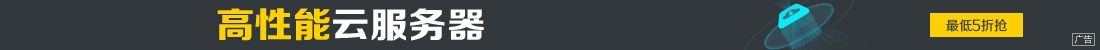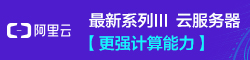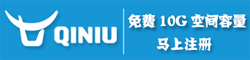# Python使用Tkinter实现滚动抽奖器效果

••Tkinter 是 Python 的标准 GUI(Graphical User Interface，图形用户接口)库，Python 使用 Tkinter 可以快速地创建 GUI 应用程序。

Tkinter 提供了非常丰富的图形界面功能，实现一个抽奖器，只需要用到里面很少的一部分功能。

1. 界面搭建2. 设置文字显示框

3. 设置按钮

```# coding=utf-8
import sys
if sys.version_info == 2:
import Tkinter
from Tkinter import *
else:
import tkinter as Tkinter
from tkinter import *
import random
data = ['风清扬', '无崖子', '东邪', '西毒', '南帝', '北丐']
going = True
is_run = False
def lottery_roll(var1, var2):
global going
show_member = random.choice(data)
var1.set(show_member)
if going:
window.after(50, lottery_roll, var1, var2)
else:
var2.set('恭喜 {} ！！！'.format(show_member))
going = True
return
def lottery_start(var1, var2):
global is_run
if is_run:
return
is_run = True
var2.set('幸运儿是你吗。。。')
lottery_roll(var1, var2)
def lottery_end():
global going, is_run
if is_run:
going = False
is_run = False
if __name__ == '__main__':
window = Tkinter.Tk()
window.geometry('405x320+250+15')
window.title('   滚 动 抽 奖 器')
bg_label = Label(window, width=70, height=24, bg='#ECf5FF')
bg_label.place(anchor=NW, x=0, y=0)
var1 = StringVar(value='即 将 开 始')
show_label1 = Label(window, textvariable=var1, justify='left', anchor=CENTER, width=17, height=3, bg='#BFEFFF',
font='楷体 -40 bold', foreground='black')
show_label1.place(anchor=NW, x=21, y=20)
var2 = StringVar(value='幸运儿是你吗。。。')
show_label2 = Label(window, textvariable=var2, justify='left', anchor=CENTER, width=38, height=3, bg='#ECf5FF',
font='楷体 -18 bold', foreground='red')
show_label2.place(anchor=NW, x=21, y=240)
button1 = Button(window, text='开始', command=lambda: lottery_start(var1, var2), width=14, height=2, bg='#A8A8A8',
font='宋体 -18 bold')
button1.place(anchor=NW, x=20, y=175)
button2 = Button(window, text='结束', command=lambda: lottery_end(), width=14, height=2, bg='#A8A8A8',
font='宋体 -18 bold')
button2.place(anchor=NW, x=232, y=175)
window.mainloop()```